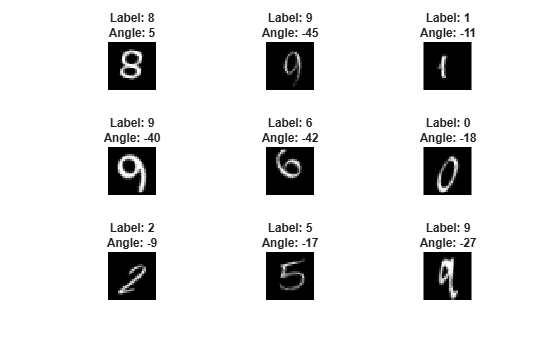Documentation

# predict

Compute deep learning network output for inference

## Syntax

``dlY = predict(dlnet,dlX)``
``[dlY1,...,dlYN] = predict(dlnet,dlX,'Outputs',layerNames)``
``[dlY1,...,dlYN,state] = predict(___)``

## Description

Some deep learning layers behave differently during training and inference (prediction). For example, during training, dropout layers randomly set input elements to zero to help prevent overfitting, but during inference, dropout layers do not change the input.

To compute network outputs for inference, use the `predict` function. To compute network outputs for training, use the `forward` function. For prediction with `SeriesNetwork` and `DAGNetwork` objects, see `predict`.

example

````dlY = predict(dlnet,dlX)` computes the network output `dlY` during inference given the input data `dlX`.```
````[dlY1,...,dlYN] = predict(dlnet,dlX,'Outputs',layerNames)` returns the outputs `dlY1`, …, `dlYN` for the specified layers.```
````[dlY1,...,dlYN,state] = predict(___)` also returns the updated network state using any of the previous syntaxes. TipFor prediction with `SeriesNetwork` and `DAGNetwork` objects, see `predict`. ```

## Examples

collapse all

This example shows how to make predictions using a `dlnetwork` object by splitting data into mini-batches.

For large data sets, or when predicting on hardware with limited memory, make predictions by splitting the data into mini-batches. When making predictions with `SeriesNetwork` or `DAGNetwork` objects, the `predict` function automatically splits the input data into mini-batches. For `dlnetwork` objects, you must split the data into mini-batches manually.

Load `dlnetwork` Object

Load a trained `dlnetwork` object and the corresponding classes.

```s = load("digitsCustom.mat"); dlnet = s.dlnet; classes = s.classes;```

Load the digits data for prediction.

```digitDatasetPath = fullfile(matlabroot,'toolbox','nnet','nndemos', ... 'nndatasets','DigitDataset'); imds = imageDatastore(digitDatasetPath, ... 'IncludeSubfolders',true);```

Make Predictions

Loop over the mini-batches of the test data and make predictions using a custom prediction loop. To read a mini-batch of data from the datastore, set the `ReadSize` property to the mini-batch size.

For each mini-batch:

• Convert the data to `dlarray` objects with underlying type single and specify the dimension labels `'SSCB'` (spatial, spatial, channel, batch).

• For GPU prediction, convert to `gpuArray` objects.

• Make predictions using the `predict` function.

• Determine the class labels by finding the maximum scores.

Specify the prediction options. Specify a mini-batch size of 128 and make predictions on a GPU if one is available. Using a GPU requires Parallel Computing Toolbox™ and a CUDA® enabled NVIDIA® GPU with compute capability 3.0 or higher.

```miniBatchSize = 128; executionEnvironment = "auto";```

Set the read size property of the image datastore to the mini-batch size.

`imds.ReadSize = miniBatchSize;`

Make predictions by looping over the mini-batches of data.

```numObservations = numel(imds.Files); YPred = strings(1,numObservations); i = 1; % Loop over mini-batches. while hasdata(imds) % Read mini-batch of data. data = read(imds); X = cat(4,data{:}); % Normalize the images. X = single(X)/255; % Convert mini-batch of data to dlarray. dlX = dlarray(X,'SSCB'); % If training on a GPU, then convert data to gpuArray. if (executionEnvironment == "auto" && canUseGPU) || executionEnvironment == "gpu" dlX = gpuArray(dlX); end % Make predictions using the predict function. dlYPred = predict(dlnet,dlX); % Determine corresponding classes. [~,idxTop] = max(extractdata(dlYPred),[],1); idxMiniBatch = i:min((i+miniBatchSize-1),numObservations); YPred(idxMiniBatch) = classes(idxTop); i = i + miniBatchSize; end```

Visualize some of the predictions.

```idx = randperm(numObservations,9); figure for i = 1:9 subplot(3,3,i) I = imread(imds.Files{idx(i)}); label = YPred(idx(i)); imshow(I) title("Label: " + label) end```## Input Arguments

collapse all

Network for custom training loops, specified as a `dlnetwork` object.

Input data, specified as a formatted `dlarray`. For more information about `dlarray` formats, see the `fmt` input argument of `dlarray`.

Layers to extract outputs from, specified as a string array or a cell array of character vectors containing the layer names.

• If `layerNames(i)` corresponds to a layer with a single output, then `layerNames(i)` is the name of the layer.

• If `layerNames(i)` corresponds to a layer with multiple outputs, then `layerNames(i)` is the layer name followed by the character "`/`" and the name of the layer output: `'layerName/outputName'`.

## Output Arguments

collapse all

Output data, returned as a formatted `dlarray`. For more information about `dlarray` formats, see the `fmt` input argument of `dlarray`.

Updated network state, returned as a table.

The network state is a table with three columns:

• `Layer` – Layer name, specified as a string scalar.

• `Parameter` – Parameter name, specified as a string scalar.

• `Value` – Value of parameter, specified as a numeric array object.

The network state contains information remembered by the network between iterations. For example, the state of LSTM and batch normalization layers.

Update the state of a `dlnetwork` using the `State` property.